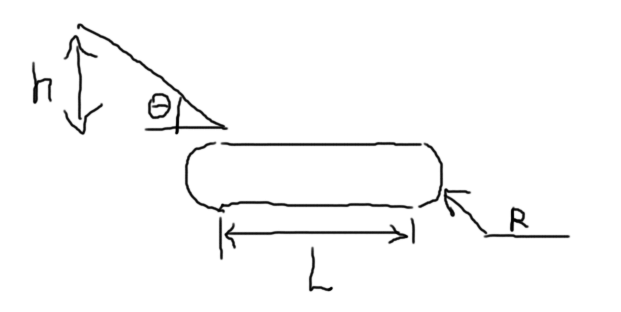2019年4月物理选考中，学考部分最后一题出现了如下的题目：$\def\dd{\mathrm d} \def\e{\mathrm e}$

• 有一个传送带，长度为 $L=2\mathrm m$ ，两侧圆弧半径为 $R=0.4\mathrm m$ ，以速度 $v>0$ 逆时针转动。左侧顶上有一个角度为 $\theta=\arcsin \frac 35\approx 37^\circ$ 的斜坡，斜坡与传送带之间的距离可以忽略，但是允许传送带从间隔处通过。斜坡高度 $h$ 处静止放置了一个小物块，物块与斜坡、与传送带的摩擦系数均为 $\mu=\frac12$
• 然后让物块自己发生滑动，落到传送带上。下落的一瞬间速度方向突变，大小不变。
• 省略无关小题，本篇注意的第二小题问：在 $h​$ 满足什么条件的时候，物块能够回到左侧、从左侧掉下。• 压力 $N​$ ，约束力，法向。
• 摩擦力 $f=-\mu N​$ ，切向。
• 重力 $mg​$ ，法向分力为 $-mg\cos\theta​$ ，切向分力为 $mg\sin\theta​$